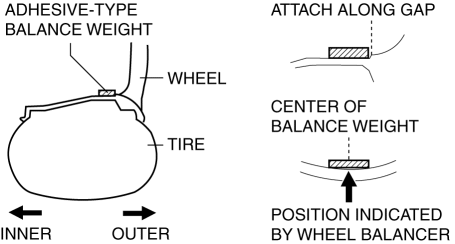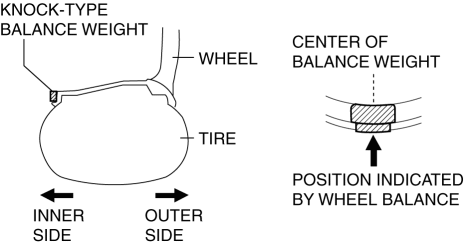Generic selectors
Exact matches only
Search in title
Search in content

# WHEEL BALANCE ADJUSTMENT (ALUMINUM ALLOY WHEEL)

< Previous Next >

## 2016 – MX-5 – Suspension

### WHEEL BALANCE ADJUSTMENT (ALUMINUM ALLOY WHEEL)

CAUTION:

• Perform the wheel balance adjustment on the outer side first, then the inner side.

• Be careful not to damage the wheel.

• Use genuine balance weight.

#### Affixing-type Balance Weight (Outer side)

1. Remove the balance weight from the wheel.

2. Remove the double-sided adhesive tape left on the wheel and remove any grease and dirt from the affixing surface.

3. Set the wheel to the wheel balancer and measure the unbalance amount and balance weight affixing position using the clip type balance weight mode.

4. Calculate the weight of the balance weight by multiplying 1.6 to the measured unbalance amount.

Example of balance weight calculation (unbalance amount displayed on wheel balancer: 23 g {0.81 oz})

• 23 g {0.81 oz}×1.6 = 36.8 g {1.30 oz}

5. Select the balance weight so that the weight is nearest to the calculated value (weight).

NOTE:

• Select the balance weight so that the weight is nearest to the calculated value.

• Ex.1) 32.4 g {1.14 oz} (calculated value) = 30 g {1.06 oz} (weight of balance weight to be selected)

• Ex.2) 32.5 g {1.15 oz} (calculated value) = 35 g {1.23 oz} (weight of balance weight to be selected)

6. Affix the selected balance weight to the balance weight affixing position (outer side) displayed by the wheel balancer.

CAUTION:

• Completely affix the balance weight by pressing it with 25 N {2.5 kgf, 5.6 lbf} or more of force per 5 g {0.2 oz} for 2 s or more.

• Do not install three or more balance weights.

• Do not affix the balance weights in parallel or stack them.

• The total weight of all the balance weights must be 160 g {5.64 oz} or less.#### Clip-type Balance Weight (Inner Side)

1. Measure the unbalance weight and the balance weight clipping point using the wheel balancer.

2. Select the balance weight equivalent to the measured unbalance amount (weight).

NOTE:

• Select the balance weight so that the weight is nearest to the calculated value.

• Ex.1) 32.4 g {1.14 oz} (calculated value) = 30 g {1.06 oz} (weight of balance weight to be selected)

• Ex.2) 32.5 g {1.15 oz} (calculated value) = 35 g {1.23 oz} (weight of balance weight to be selected)

3. Install the selected balance weight to the balance weight clipping position (inner side) displayed on the wheel balancer.

CAUTION:

• Do not install three or more balance weights.

• The weight of one balance weight must be 60 g {2.1 oz} or less, and the total weight of two balance weights must be 100 g {3.53 oz} or less.#### Residual Unbalance Amount Verification

1. After installing the balance weights on the outer and inner sides, measure the wheel balance again. Standard

 Outer side (affixing type) Inner side (clip type) 16 inch wheel 14 g {0.49 oz} 9 g {0.3 oz} 17 inch wheel 13 g {0.46 oz} 8 g {0.3 oz}

• If not within the specification, adjust the wheel balance again.

© 2014 Mazda North American Operations, U.S.A.